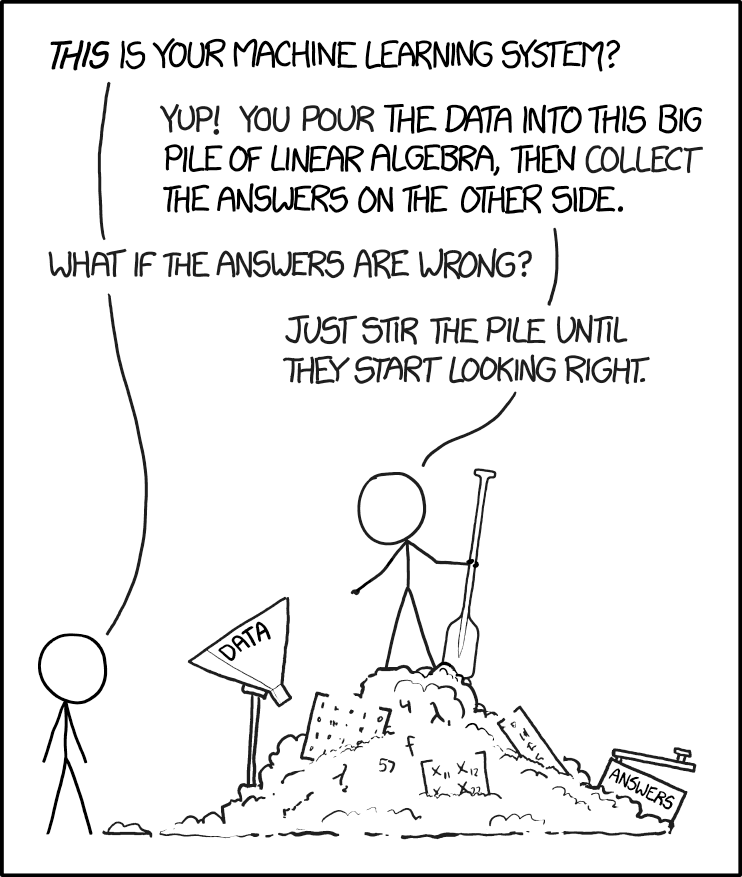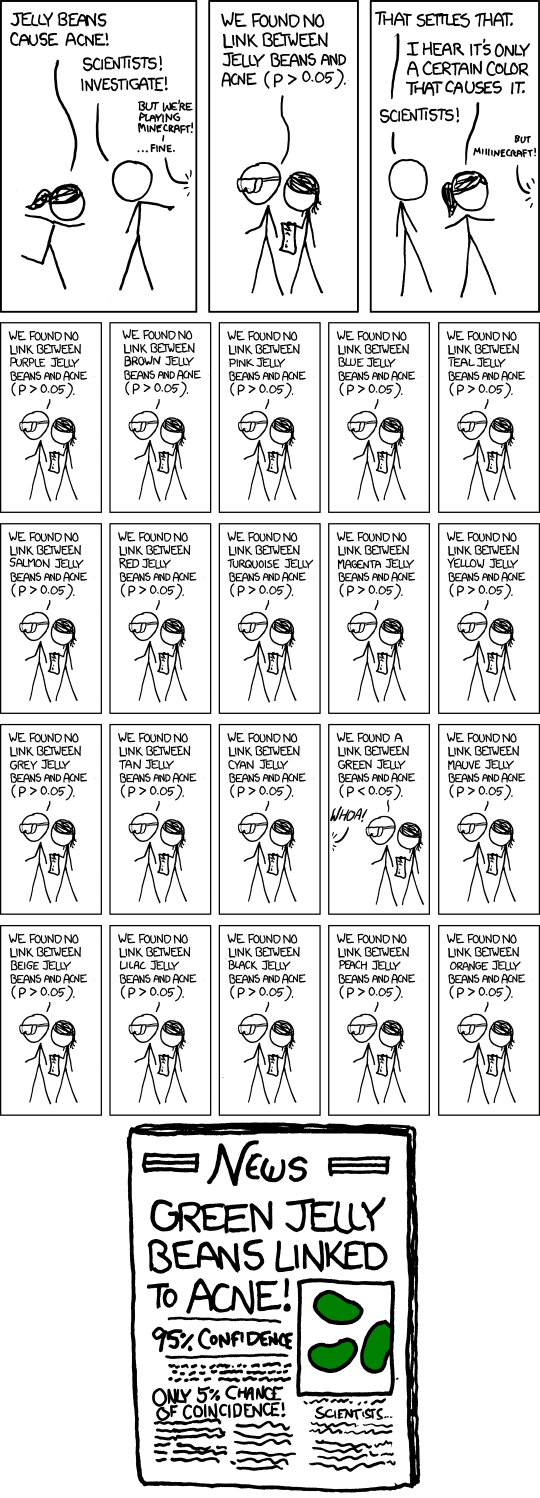## Stirring the pile efficiently

Warning: This is a technical post, and involves some code, etc.

As I’ve ranted a fair bit on this blog over the last year, a lot of “machine learning” in the industry can be described as “stirring the pile”. Regular readers of this blog will be familiar with this image from XKCD by now:Source: https://xkcd.com/1838/

Basically people simply take datasets and apply all the machine learning techniques they have heard of (implementation is damn easy – scikit learn allows you to implement just about any model in three similar looking lines of code; See my code here to see how similar the implementation is).

So I thought I’ll help these pile-stirrers by giving some hints of what method to use for different kinds of data. I’ve over-simplified stuff, and so assume that:

1. There are two predictor variables X and Y. The predicted variable “Z” is binary.
2. X and Y are each drawn from a standard normal distribution.
3. The predicted variable Z is “clean” – there is a region in the X-Y plane where Z is always “true” and another region where Z is always “false”
4. So the idea is to see which machine learning techniques are good at identifying which kind of geometrical figures.
5. Everything is done “in-sample”. Given the nature of the data, it doesn’t matter if we do it in-sample or out-of-sample.

For those that understand Python (and every pile-stirrer worth his salt is excellent at Python), I’ve put my code in a nice Jupyter Notebook, which can be found here.

So this is what the output looks like. The top row shows the “true values” of Z. Then we have a row for each of the techniques we’ve used, which shows how well these techniques can identify the pattern given in the top row (click on the image for full size).As you can see, I’ve chosen some common geometrical shapes and seen which methods are good at identifying those. A few pertinent observations:

1. Logistic regression and linear SVM are broadly similar, and both are shit for this kind of dataset. Being linear models, they fail to deal with non-linear patterns
2. SVM with RBF kernel is better, but it fails when there are multiple “true regions” in the dataset. At least it’s good at figuring out some non-linear patterns. However, it can’t figure out the triangle or square – it draws curves around them, instead.
3. Naive Bayesian (I’ve never understood this even though I’m pretty good at Bayesian statistics, but I understand this is a commonly used technique; and I’ve used default parameters so not sure how it is “Bayesian” even) can identify some stuff but does badly when there are disjoint regions where Z is true.
4. Ensemble methods such as Random Forests and Gradient Boosting do rather well on all the given inputs. They do well for both polygons and curves. Elsewhere, Ada Boost mostly does well but trips up on the hyperbola.
5. For some reason, Lasso fails to give an output (in the true spirit of pile-stirring, I didn’t explore why). Ridge is again a regression method and so does badly on this non-linear dataset
6. Neural Networks (Multi Layer Perceptron to be precise) does reasonably well, but can’t figure out the sharp edges of the polygons.
7. Decision trees again do rather well. I’m pleasantly surprised that they pick up and classify the disjoint sets (multi-circle and hyperbola) correctly. Maybe it’s the way scikit learn implements them?

Of course, the datasets that one comes across in real life are never such simple geometrical figures, but I hope that this set can give you some idea on what techniques to use where.

At least I hope that this makes you think about the suitability of different techniques for the data rather than simply applying all the techniques you know and then picking the one that performs best on your given training and test data.

That would count as nothing different from p-hacking, and there’s an XKCD for that as well!Source: https://xkcd.com/882/